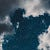# Using Artificial Neural Network for classification to solve Machine learning problem-Part 1(EDA)

Just recently I started learning Deep Learning, the very first algorithm I learned is Artificial Neural Network, so we will be using ANN to solve machine learning problem and in the end we will check accuracy of ANN and ML algorithm to see which one is better.

We will try to solve the following problem:

A company which is active in Big Data and Data Science wants to hire data scientists among people who successfully pass some courses which are conducted by the company. Many people sign up for their training. The company wants to know which of these candidates…

# Random Forest(🎄🌴🌲) for Regression…

Random Forest or Random Decision Forest, is a machine learning algorithm. It is also one of the most used algorithms, because of its simplicity.

Random forest is an ensemble method that trains several decision trees using the “bagging” method.

Before understanding Random Forest intuition, let’s understand

## Why, Random Forest?

Random Forest is a replacement for the Decision tree. Like a forest, the Random Forest algorithm consists of multiple single decision trees. It decreases the variance of the model without increasing the bias. It is also great with the higher dimensional data and faster to train than the decision tree.

Fact: Random Forest are…

# Decision Tree🌳 using Python(for Regression)

The decision tree is the most commonly used algorithm in the practice, the reason behind is that it can be used in Regression and classification.

A decision tree is a decision support tool that uses a tree-like model of decisions and their possible consequences, including chance event outcomes, resource costs, and utility. It is one way to display an algorithm that only contains conditional control statements.

I will provide a link to my Kaggle notebook in the end.

Now let’s code!!!

## 1. Dataset:

The dataset is related to red and white variants of the Portuguese “Vinho Verde” wine. It has twelve columns…

# Support Vector Regression using Python

Usually, Most of us get confused between support vector machine(SVM) and support vector regression(SVR). Well, the basic difference is that SVM is used in the classification, and SVR is used in the regression.

What exactly is an SVR? To understand what SVR is we are going to compare it with the linear regression.

As we all know, in linear regression we draw the linear line through the data points, then we project a vertical projection of the data points on the regression line to find the minimum distance.

We will do something like that but instead of drawing only the…

# Polynomial Regression in Python

When your linear regression algorithm(straight line through dataset) fails to fit the data points then it might be ideal to use Polynomial Regression(PR).

## Polynomial Regression:

Polynomial regression is the nonlinear relationship between an independent variable(X) and a dependent variable(y), and it is modeled as nth degree polynomial in X.

Question: If it is a nonlinear then why is it called as polynomial linear regression?

Answer: It is the form of linear regression. In every linear regression equation to find the value of dependent variable(y) we first need to find the values of coefficient(b1, b2, …,bn) and interceptor(b0). For any values of independent…

# Multiple Linear Regression using Python

In Linear regression, the most common way to train your model is by using Multiple Linear Regression(MLR). Before start coding our model, let’s understand more about MLR.

## Linear Regression:

As noun sounds, Linear Regression is a linear approach to predict the relationship between independent and dependent variables.

## Multiple Linear Regression:

MLR is also a linear approach, but instead of using a single independent variable like simple linear regression, we will use multiple independent variables with one dependent variable.

I guess now you know a little bit more about multiple linear regression. I will divide this article into seven different coding stages,

1. Importing the libraries
2. Importing…

# Simple Linear Regression Model using Python

The very first step in ML is the Simple Linear Regression model, so let’s get started.

Before learning about simple linear regression, let’s understand what is Regression and then what is Linear regression.

## Regression:

In simple words, our problem is a regression problem when the output variable is real or continues value, such as ‘salary’, ‘price’ or ‘weight’.

## Linear Regression:

Linear regression is a linear approach to modeling the relationship between independent and dependent variables.

## Simple Linear Regression:

SLR is a linear approach to modeling the relationship between a single independent and dependent variable.

Now you know what SLR is, let’s code it using python language.

# How to start learning Machine Learning?

After giving a lot of thought to whether you want to be a machine learning engineer or not, you decided to become one. Now I am not going to say that if you decide anything to do then half of your work is already done, no that is so not true. There are lots of things to learn in machine learning, and you are probably going to get distracted, confused, lost, and maybe something more. Cause it happened with me, with my friends, and mostly with all the other machine learning engineers. I know, I know we learn more from…